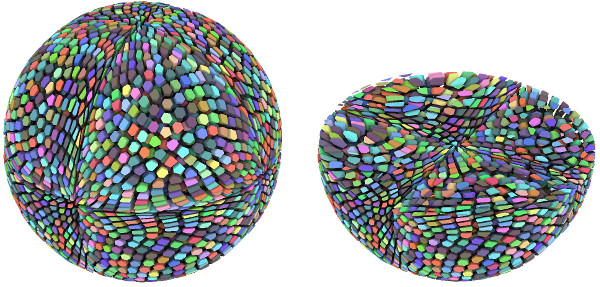ANR project MAGA

ANR project MAGA

The MAGA project is funded by the French ANR for four years (2016—2020). The acronym MAGA stands for Monge-Ampère et Géométrie Algorithmique.

Description

The Monge-Ampère equation is a fully nonlinear elliptic equation, which plays a central role in geometry and in the theory of optimal transport. However, the singular and non-linear nature of the equation is a serious obstruction to its efficient numerical resolution. The first aim of the MAGA project is to study and to implement discretizations of optimal transport and Monge-Ampère equations which rely on tools from computational geometry (Laguerre diagrams). In a second step, these solvers will be applied to concrete problems from various fields involving optimal transport or Monge-Ampère equations such as

• computational physics: early universe reconstruction problem, design of Lagrangian schemes handling congestion/incompressibility constraints
• economics: principal agent problems, urban planning, optimal pits design
• geometry: variational problems over convex bodies, reflector and refractor design for non-imaging opticsLaguerre diagram corresponding to the optimal transport between uniform measures over the unit ball and the so-called "tangle cube".

Fluid simulations using semi-discrete optimal transport.

Members

• Université Paris-Sud / Polytechnique
• Yann Brenier, École Polytechnique/CNRS
• Bertrand Maury, Université Paris-Sud
• Quentin Mérigot, Université Paris-Sud (coordinator)
• Luca Nenna, Université Paris-Sud
• Jean-Marie Mirebeau, Université Paris-Sud/CNRS
• Filippo Santambrogio, Université Lyon 1
• Université Paris-Dauphine / CNRS délégation régionale Paris B / Inria Paris
• Jean-David Benamou, Inria Paris
• Thomas Gallouet, Inria Paris
• Guillaume Carlier, Université Paris-Dauphine (local coordinator)
• François-Xavier Vialard, Université Paris-Est
• Université Grenoble-Alpes / Grenoble INP / Inria Nancy
• Dominique Attali, Grenoble-INP/CNRS
• Bruno Lévy, Inria Nancy
• Jocelyn Meyron, Grenoble-INP/Université Grenoble-Alpes
• Édouard Oudet, Université Grenoble-Alpes
• Boris Thibert, Université Grenoble-Alpes (local coordinator)